﻿ A New Approach for Introducing Schrödinger’s Equation Using Maxwell’s Equations, Quantum Mechanics, and Special RelativityPublications are Open
Access in this journal
Article Versions
Export Article
• Normal Style
• MLA Style
• APA Style
• Chicago Style
Original Article
Open Access Peer-reviewed

### A New Approach for Introducing Schrödinger’s Equation Using Maxwell’s Equations, Quantum Mechanics, and Special Relativity

Hye Jung Kang
American Journal of Educational Research. 2018, 6(7), 963-966. DOI: 10.12691/education-6-7-11
Published online: July 10, 2018

### Abstract

The wave-particle duality for light has been well established by various experiments, such as Young’s double slit experiment and the photoelectric effect. This led de Broglie to propose that a particle also has wave characteristics. Schrödinger further established the wave equation for a moving particle. Many times, quantum mechanics textbooks do not show how Schrödinger’s equation was developed in an intuitive manner that is appropriate at the undergraduate level. This article presents a new approach for introducing Schrödinger’s equation. This new approach starts with Maxwell’s equations and then applies the quantized energy of a light wave and special relativity. This more intuitive approach should help undergraduate students understand the origin of Schrödinger’s equation in a more natural way.

### 1. Introduction

Quantum mechanics is one the most revolutionary theories in modern science. It is now applied to many areas of science and technologies; physics, chemistry, biology, and numerous electronic devices. Quantum mechanics successfully describes both the wave characteristics and the particle characteristics of light and moving particles.

The core concept of quantum mechanics starts with the wave-particle duality for light, which has been well established by various experiments, such as Young’s double slit experiment and the photoelectric effect. To explain the Planck’s blackbody radiation and the photoelectric effect, Einstein proposed that the energy of a light wave is quantized. This quantized energy of a light wave is called a photon, which also shows the particle characteristics of light.

De Broglie proposed that a moving particle may have this wave-particle duality 1. Schrödinger further established the wave equation for a moving particle 2. Schrödinger’s equation predicts the behavior of particles in small scale very accurately. However, many times, quantum mechanics textbooks do not show how Schrödinger’s equation was developed in an intuitive manner that is appropriate at the undergraduate level.

This article presents a new approach for introducing Schrödinger’s equation for undergraduate education. First, it will derive the wave equation for a light wave from Maxwell’s equations. The solution of the wave equation for a light wave is a function of the frequency and the wave function. Second, to associate the solution of the wave equation for a light wave with the particle properties, it will express the frequency in terms of energy of a photon and the wave function in terms of momentum of a photon using the special relativity. Third, it will show that the energy and the momentum of a photon can be expressed in partial differential forms, called operators. By symmetry, it is very reasonable to assume that a particle can have the wave-particle duality. Therefore, last, it will establish a wave equation for a moving particle by expressing the energy and the momentum of a particle with their operators. This article also explores the current interpretation of the wave function.

This new approach for introducing Schrödinger’s equation of a particle should help students understand the origin of Schrödinger’s equation in a more natural way.

### 2. A New Approach for Introducing Schrödinger’s Equation

2.1. Wave Equation for Light

Maxwell’s equations in a vacuum are described as 3,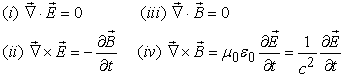(1)

Here,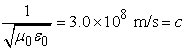is the speed of light. The decoupled differential equation for the electric field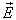can be obtained by taking the curl to (ii) in equation (1) 3.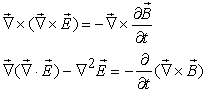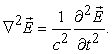(2)

From (i) and (iv) in equation (1),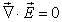and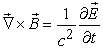are used.

Equation (2) is a wave equation formoving at the speed of light c. Similarly, the wave equation for the magnetic field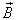can be obtained by taking the curl to (iv) in equation (1),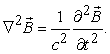(3)

Wave equations (2) and (3) imply that light is a propagation of electromagnetic waves moving at speed c. Note that (ii) in equation (1) says that the electric field and the magnetic field are perpendicular to each other. For a plane light wave moving along the +x axis with the electric field pointing along the z axis, the electric field can be described as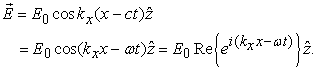(4)

Here, Euler’s formula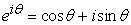is used and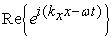denotes a real part of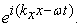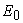is the amplitude of the electric field.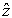is a unit vector along the z axis. The wave vector for a wave moving along the +x axis is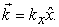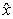is a unit vector along the x axis. Its amplitude (wave number) is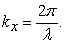Therefore, the relation below is used in equation (4)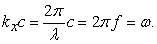(5)

The angular frequency ω can be expressed with the energy E of a light wave. From the photoelectric effect, the quantized energy of a light wave (photon) with frequency f is expressed as,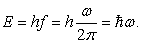(6)

Here,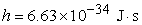is Planck’s constant. h-bar is defined as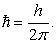According to the special relativity, the energy of a photon can be written as 4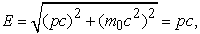(7)

since the rest mass of a photon is zero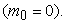From equations (5), (6) and (7), the wave vector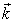of light can be expressed with its momentum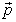as,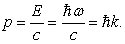(8)

Equation (8) is de Broglie's formula that relates the momentum with the wavelength;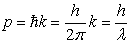1.

From equations (6) and (8), the electric field of a light wave in equation (4) can be written as,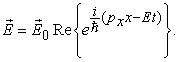(9)

The next step is to find a way to associate the momentum of light with one of the partial derivatives in the wave equation (2). Taking the partial derivative ofin equation (9) with respect to x gives,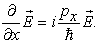(10)

The above differential equation is an eigenvalue equation. When the partial derivative with respect to position x is applied to a function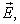it gives a functionitself multiplied by a value. Since this value is associated with the momentum, this partial derivative is called an operator for the momentum. From equation (10), the operator for the momentum can be expressed as,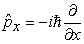(11)

Here, the hat notation is used to indicate that it is an operator. The general form of equation (11) for the three dimensional case is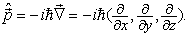(12)

Similarly, we can associate the energy of light with the other partial derivative in the wave equation (2). Taking the partial derivative ofin equation (9) with respect to time t gives,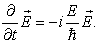Now, the operator for the energy can be expressed as,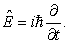(13)

When equations (12) and (13) are applied to the wave equation for light in equation (2), it gives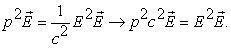(14)

The wave equation (2) for a photon produces the correct relation between the energy of a photon and its momentum shown in equation (7).

2.2. Wave equation for a Moving Particle

The wave-particle duality of light is well described by the wave equation for light when the momentum and the energy of a photon are expressed using operators. By symmetry, it is reasonable to assume that a particle also has this wave-particle duality.

Schrödinger used this idea to describe a moving particle. He introduced a wave function Ψ that plays a similar role as the electric field and the magnetic field for light. He also derived a wave equation for Ψ, called Schrödinger’s equation 2. However, his original derivation is beyond the undergraduate level.

This article introduces Schrödinger’s equation in an intuitive manner that is appropriate at the undergraduate level. In section 2.1, we found that the wave equation for light can be obtained by applying operators of energy and momentum to a function. Therefore, Schrödinger’s equation for a moving particle can also be obtained by applying operators of energy and momentum to the wave function Ψ(x,y,z,t).

Since the total energy of a moving particle is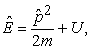it can be written as an operator form by using equations (12) and (13),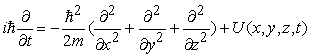By applying the operator above to the wave function, the wave equation for a moving particle can be written as,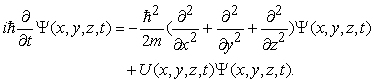(15)

This is Schrödinger’s equation for a moving particle that is analogous to the wave equation for light in equation (2).

2.3. Interpretation of the Wave Function

Now, let’s consider the meaning of the wave function Ψ. First, to have a physical meaning, the wave function should have a real value. Note that the solution of the wave equation for light,is a physically measurable quantity and thus, it has a real value. Since the wave function Ψ is complex, by itself, it does not have any physical meaning. One way to have a real value is to multiply Ψ with its complex conjugate.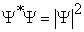has a positive real value and thus it has a physical meaning.

To give a physical meaning to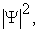we compare its role with the role of the electric fieldfor light. The energy of light is associated with its frequency as shown in equation (6). This means that light with the same color has the same energy regardless of its brightness (intensity). Brighter light of the same color has more photons and this implies a higher probability of finding a photon at a given location. Since the intensity of light is proportional to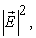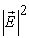is a measure of the probability density (a probability per unit volume) of finding a photon at a location 5.

Similarly, we can interpret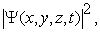as a probability density of finding a particle at a given position and a given time. Since a particle should be found somewhere, the probability of finding a particle in space should be one. Therefore, one of the conditions for the wave function is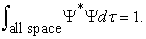(16)

Second, there is a set of wavefunctions that satisfies Schrödinger’s equation. Each wavefunction in a set is called a basis function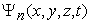and it represents a possible particular state n that a particle can be. A wave function is written as a linear combination of these basis functions,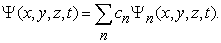(17)

Here, cn is an expansion coefficient and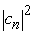is a probability of a particle being in the state n. The average value of energy E that we would measure would be 6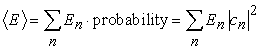(18)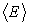denotes the average value of E and it is called the expectation value in quantum mechanics. Note that equation (17) is similar to a musical note that is composed of simple sinusoidal waves with different frequencies.

Last, what is the role of the wavefunction Ψ if it does not have a physical meaning? Although it cannot be measured, it contains all information about possible outcomes of all measurements. The physically measurable values, such as energy and momentum of a particle, can be obtained by applying the corresponding operators to the wave function. For example, the energy of a particle in the state n can be obtained by applying the energy operator to its basis wave function Ψn.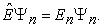(19)

Here, En is the energy of state n. After a measurement, the state of a particle changes. The Schrödinger’s equation shown in equation (15) describes the evolution of the wave function as a function of time.

### 3. Conclusion

This article introduced Schrödinger’s equation by starting with Maxwell’s equations and then applying the quantized energy of a light wave and special relativity.

First, a wave equation for light is derived from Maxwell’s equations. Then, the solution of the wave equation for light is expressed in terms of energy and momentum using the quantized energy of a light wave and Einstein’s special relativity. In this process, it is found that measurable values of energy and momentum can be expressed in partial differential forms, called operators. By analogy, the wave equation for a moving particle is also established by applying the wave-particle duality to a particle. I think that this approach can help students understand the origin of Schrödinger’s equation in a more natural way. This article also intuitively demonstrates the current interpretation of the wave function.

### References

  De Broglie, L., The wave nature of the electron, Nobel Lecture, 1929, Physics 1922-1941, Elsevier Publishing Company, Amsterdam, 1965, 244-256. In article  Schrödinger, E., “An undulatory theory of the mechanics of atoms and molecules,” Physical Review, 28 (6). 1049-1070. Dec. 1926. In article View Article  Griffiths, D. J., Introduction to Electrodynamics, 4th ed, Pearson, 2013, 393-394. In article View Article  Helliwell, T. M., Special Relativity, University Science Books, 2010, 146-150. In article  Giancoli, D. C., Physics for Scientists & Engineers with Modern Physics, 4th ed, Pearson Prentice Hall, Upper Saddle River, 2009, 1018-1019. In article View Article  Miller, D. A., Quantum Mechanics for Scientists and Engineers, Cambridge University Press, New York, 2008, 73-74. In article View ArticleThis work is licensed under a Creative Commons Attribution 4.0 International License. To view a copy of this license, visit http://creativecommons.org/licenses/by/4.0/